# 【神经网络搜索】Efficient Neural Architecture Search¶

【GiantPandaCV导语】本文介绍的是Efficient Neural Architecture Search方法，主要是为了解决之前NAS中无法完成权重重用的问题，首次提出了参数共享Parameter Sharing的方法来训练网络，要比原先标准的NAS方法降低了1000倍的计算代价。从一个大的计算图中挑选出最优的子图就是ENAS的核心思想，而子图之间都是共享权重的。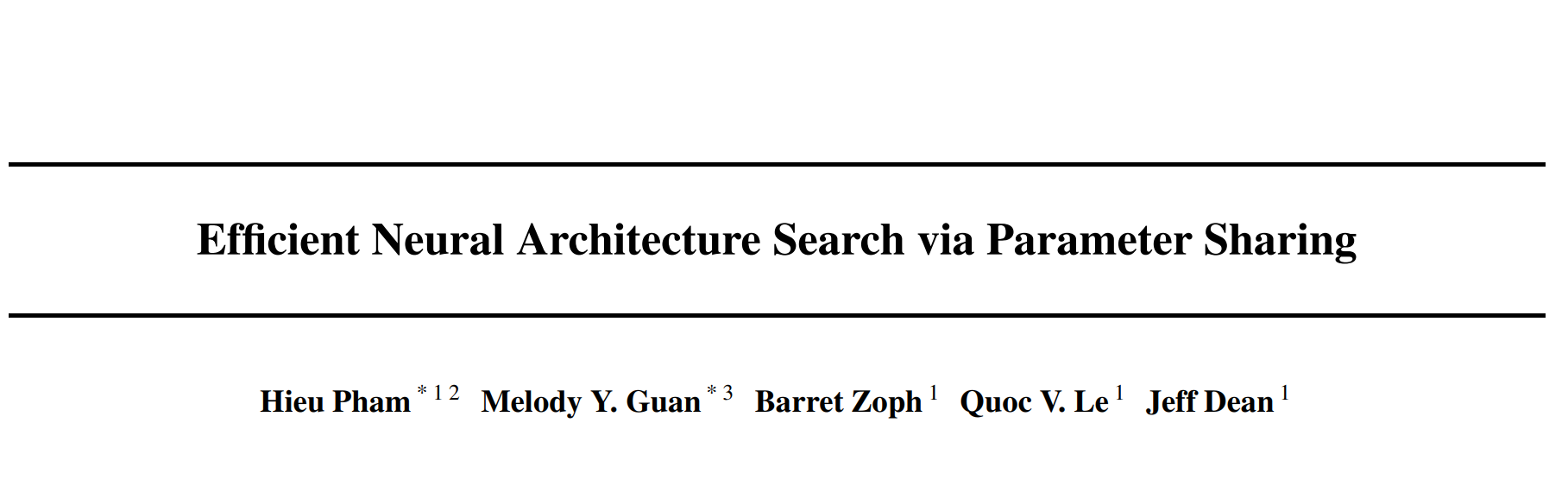## 1. 摘要¶

ENAS是一个快速、代价低的自动网络设计方法。在ENAS中，控制器controller通过在大的计算图中搜索挑选一个最优的子图来得到网络结构。

• 被挑选的子图将使用经典的CrossEntropy Loss进行训练。

## 2. 简介¶

NAS-RL使用了450个GPU训练了3-4天，花费了32,400-43,200个GPU hours才可以训练出一个合适的网络，需要大量的计算资源。NAS的计算瓶颈就在于需要让每个子模型从头开始收敛，训练完成后就废弃掉其训练好的权重。

ENAS可以做到使用单个NVIDIA GTX 1080Ti显卡，只需要花费16个小时。同时在CIFAR10上可以达到2.89%的test error。

## 3. 方法¶

### 3.1 一个例子¶

ENAS可以看作是从一个超网中得到一个自网络，如下图所示。6个节点相互连接得到的就是超网（是一个有向无环图），通过controller得到红色的路径就是其中的一个子网络。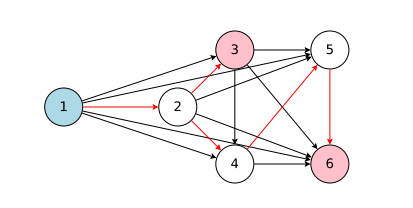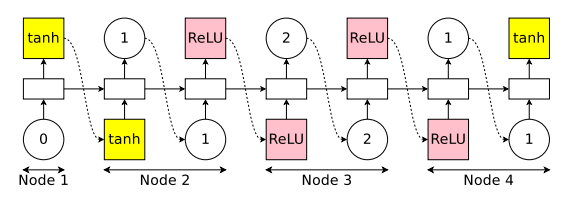• 激活哪个边
• 对应Node选择什么操作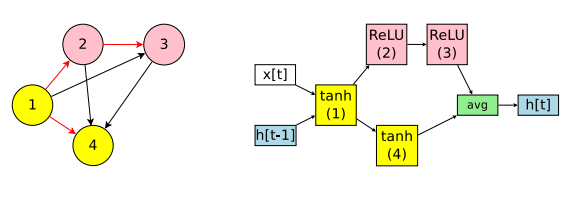### 3.2 ENAS训练流程¶

• LSTM sample出一个子模型，然后训练模型$w$， 通过标准的反向传播算法进行训练，训练完成以后在验证集上进行测试。
• 通过验证集上结果反馈给LSTM，计算$\theta$的梯度，更新LSTM的参数。
• 如此反复，可以训练出一个LSTM能够让模型在验证集上的性能最佳。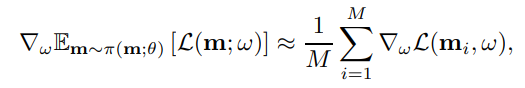m是从$\pi(m;\theta)$ 中采样得到的模型，对于所有的模型计算模型损失函数的期望。右侧公式是梯度的无偏估计。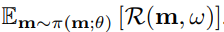### 3.3 marco search space¶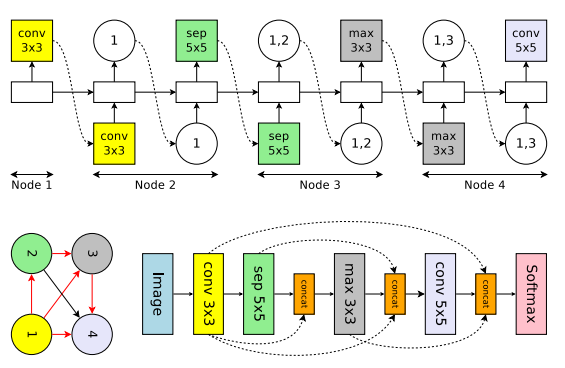• 节点操作不同，这里可以是3x3卷积、5x5卷积、平均池化、3x3最大池化、3x3深度可分离卷积，5x5深度可分离卷积 一共六个操作。
• 上图Node3输出了两个值，代表先将node1和node2的输出tensor合并，然后在经过maxpool操作。

### 3.4 micro search space¶

ENAS中首次提出了搜索一个一个单元，然后将单元组合拼接成整个网络。其中单元分为两种类型，一种是Conv Cell 该单元不改变特征图的空间分辨率；另外一种是Reduction Cell 该单元会将空间分辨率降低为原来的一半。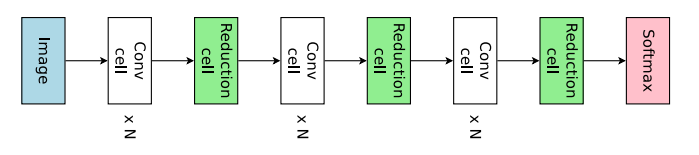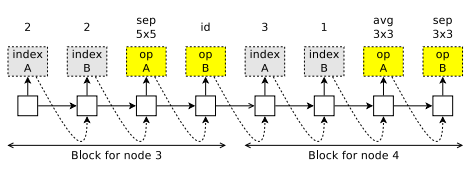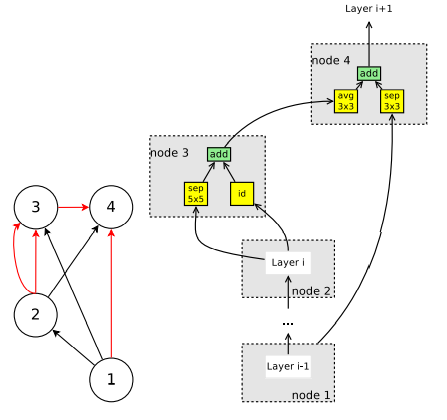## 4. 实验结果¶

### 4.1 语言模型¶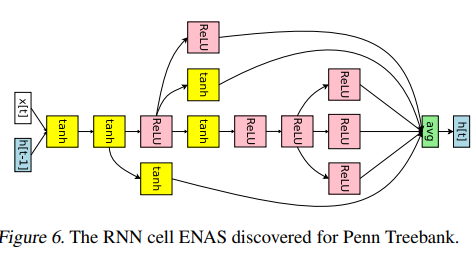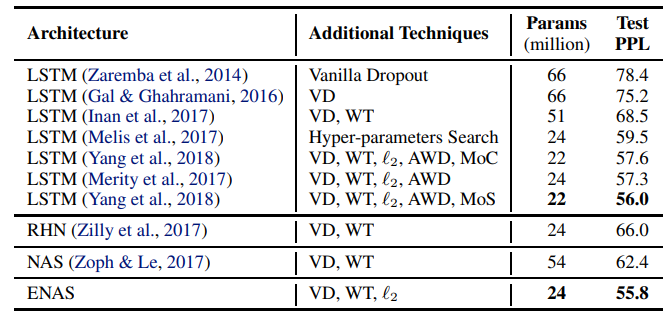### 4.2 图像分类¶

controller的设置细节，policy gradient的权重$\theta$使用均匀的从[-0.1，0.1]初始化，使用0.00035的学习率,使用Adam优化器，设置tanh常数为2.5 temerature 设置为5.0; 给controller 得到的熵添加0.1的权重。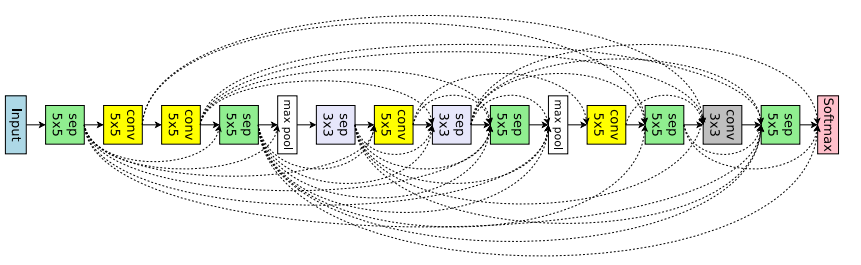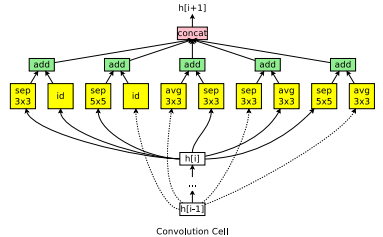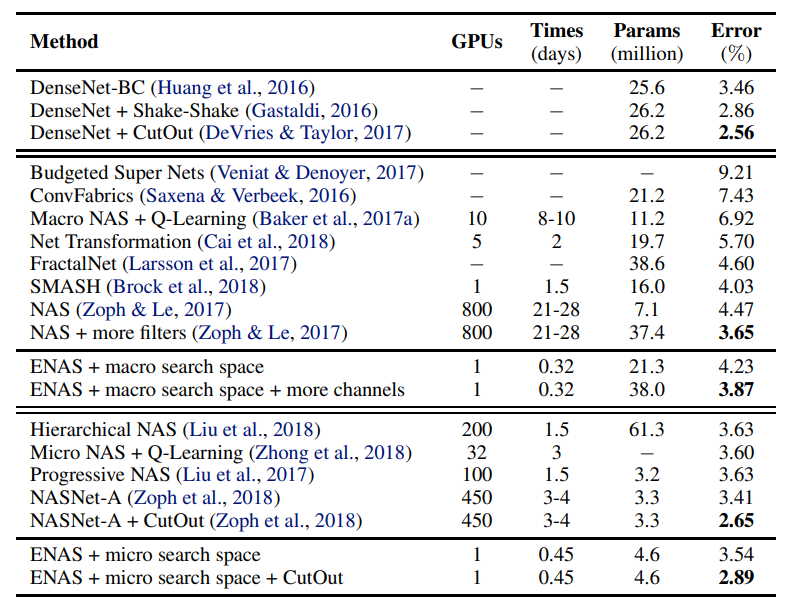## 5. 代码实现¶

class ENASLayer(mutables.MutableScope):

def __init__(self, key, prev_labels, in_filters, out_filters):
super().__init__(key)
self.in_filters = in_filters
self.out_filters = out_filters

self.mutable = mutables.LayerChoice([
ConvBranch(in_filters, out_filters, 3, 1, 1, separable=False),
ConvBranch(in_filters, out_filters, 3, 1, 1, separable=True),
ConvBranch(in_filters, out_filters, 5, 1, 2, separable=False),
ConvBranch(in_filters, out_filters, 5, 1, 2, separable=True),
PoolBranch('avg', in_filters, out_filters, 3, 1, 1),
PoolBranch('max', in_filters, out_filters, 3, 1, 1),
SEConvBranch(in_filters, out_filters, 3, 1, 1, reduction=4)
])
if len(prev_labels) > 0:
self.skipconnect = mutables.InputChoice(
choose_from=prev_labels, n_chosen=None)
else:
self.skipconnect = None
self.batch_norm = nn.BatchNorm2d(out_filters, affine=False)

def forward(self, prev_layers):
out = self.mutable(prev_layers[-1])
if self.skipconnect is not None:
connection = self.skipconnect(prev_layers[:-1])
if connection is not None:
out += connection
return self.batch_norm(out)


• mutable LayerChoice就是从备选选项中选择其中一个操作
• mutable InputChoice是选择前几层节点进行连接。

class GeneralNetwork(nn.Module):
def __init__(self, num_layers=6, out_filters=12, in_channels=3, num_classes=10,
dropout_rate=0.0):
super().__init__()
self.num_layers = num_layers
self.num_classes = num_classes
self.out_filters = out_filters
self.dropout_rate = dropout_rate

self.stem = nn.Sequential(
nn.Conv2d(in_channels, out_filters, 3, 1, 1, bias=False),
nn.BatchNorm2d(out_filters)
)

pool_distance = self.num_layers // 3
# 进行pool操作是num_layers // 3
self.pool_layers_idx = [pool_distance - 1, 2 * pool_distance - 1]
self.dropout = nn.Dropout(self.dropout_rate)

self.layers = nn.ModuleList()  # convolutional
self.pool_layers = nn.ModuleList()  # reduction

labels = []
for layer_id in range(self.num_layers):  # 设置12个layer
labels.append("layer_{}".format(layer_id))

if layer_id in self.pool_layers_idx:  # 如果使用pool
self.pool_layers.append(FactorizedReduce(
self.out_filters, self.out_filters))

self.layers.append(  # 相当于Node节点
ENASLayer(labels[-1], labels[:-1], self.out_filters, self.out_filters))

self.dense = nn.Linear(self.out_filters, self.num_classes)

def forward(self, x):
bs = x.size(0)
cur = self.stem(x)

layers = [cur]

for layer_id in range(self.num_layers):
cur = self.layers[layer_id](layers)
layers.append(cur)
if layer_id in self.pool_layers_idx:
# 如果轮到了池化层
for i, layer in enumerate(layers):
layers[i] = self.pool_layers[self.pool_layers_idx.index(
layer_id)](layer)
cur = layers[-1]

cur = self.gap(cur).view(bs, -1)
cur = self.dropout(cur)
logits = self.dense(cur)
return logits


• self.stem是第一个node，手动设置的。
• 池化是强制设置的，在某些层规定进行下采样。

model = GeneralNetwork()
trainer = enas.EnasTrainer(model,
loss=criterion,
metrics=accuracy,
reward_function=reward_accuracy,
optimizer=optimizer,
callbacks=[LRSchedulerCallback(lr_scheduler), ArchitectureCheckpoint("./checkpoints")],
batch_size=args.batch_size,
num_epochs=num_epochs,
dataset_train=dataset_train,
dataset_valid=dataset_valid,
log_frequency=args.log_frequency,
mutator=mutator)


mutator是NNI提供的一个类，就是上述提到的controller，这里具体调用的是EnasMutator。

def _sample_layer_choice(self, mutable):
# 选择 某个层 只需要选一个就可以了
self._lstm_next_step() # 让_inputs在lstm中进行一次前向传播

logit = self.soft(self._h[-1]) # linear 从隐藏层embedd得到可选的层的逻辑评分

if self.temperature is not None:
logit /= self.temperature # 一个常量 貌似是RL中的trick

if self.tanh_constant is not None:
# tanh_constant * tanh(logits) 用tanh再激活一次（可选）
logit = self.tanh_constant * torch.tanh(logit)

if mutable.key in self.bias_dict:
logit += self.bias_dict[mutable.key]
# 对卷积层进行了偏好处理，如果是卷积层，那就在对应的值加上一个0.25，增大被选中的概率

# softmax, view(-1),
branch_id = torch.multinomial(F.softmax(logit, dim=-1), 1).view(-1)
# 依据概率来选下角标，如果数量不为1，选择的多个中没有重复的
# eg: [100,1,1] 最有可能选择100对应的下标0

log_prob = self.cross_entropy_loss(logit, branch_id) # 交叉熵损失函数 - 判断logit和branchid分布是否相似程度

self.sample_log_prob += self.entropy_reduction(log_prob) # 求和或者求平均

entropy = (log_prob * torch.exp(-log_prob)).detach()  # pylint: disable=invalid-unary-operand-type　??

self.sample_entropy += self.entropy_reduction(entropy) # 样本熵？

self._inputs = self.embedding(branch_id) # 得到对应id的embedding, 从选择空间 - 映射到 - 隐空间

return F.one_hot(branch_id, num_classes=self.max_layer_choice).bool().view(-1) # 将选择变成one_hot向量


if self.entropy_weight: # 交叉熵权重
reward += self.entropy_weight * self.mutator.sample_entropy.item() # 得到样本熵


## 6. 总结¶

ENAS核心就是提出了一个超网，每次从超网中采样一个小的网络进行训练。所有的子网络都是共享超网中的一套参数，这样每次训练就不是从头开始训练，而是进行了迁移学习，加快了训练速度。